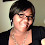## Thursday, November 14, 2013

### Graphically Analyzing Inequalities and Equations Flexibly

I've been doing inequalities and equations in the coordinate plane with my Algebra 2 kids, and I just love it. I see extensions from this topic to lots of others.

We started by looking at the meaning of <, >, and = inside the coordinate plane. We established quickly that when two things are equal in the coordinate plane, they must overlap. For example, (6, 2)= (6, 2) because they overlap. Also, y = x + 3 is equal to y = x + 3 because they overlap.

Then, we discussed that < means "lower than" and > means "higher than."

For any given equation or inequality, we would graph it as Y1 and Y2.

For example, 2x + 3 = 3x - 8, means that Y1=2x + 3 has to overlap with Y2=3x - 8. The kids sketch the graph, label the intersection, and answer in terms of x: x = 11. Easy breezy.

For inequalities, the kids learn to first analyze them using graphs before reviewing how to solve them by hand. For example, 2x + 3 < 3x - 8, means that Y1=2x + 3 has to be lower than Y2=3x - 8. The kids sketch the graph, label the intersection, and draw a vertical dashed line at x = 11 to separate the plane into two sections. They put their hands to cover one of the halves of the plane and ask, "Is Y1 actually lower than Y2 here?" If so, they shade it.

It looks something like this:

and from that, the kids can conclude that the solutions for x are all to the right of x = 11, so they write down x > 11.

On the quiz for inequalities, my kids had 100% accuracy on all the equations and inequality questions because they were asked to show their work two ways, one by hand and one by the graph, for each equation or inequality. They needed to get the same answer using both methods, in order to gain full reflection points (as well as accuracy and process points). Most of the kids were also able to explain why two parallel lines would cause an equation to have no solution, and inequalities to have either infinite solutions or no solutions, depending on the direction of the inequality symbol.

What I love about this graphical emphasis is how extendable this is to different topics. Today in one class I asked the kids to solve -20 < -4x + 5 < 11, and half the class decided to solve it by hand and the other half decided to solve it by just looking at the graph. They determined that only the middle part of the graph satisfies the inequality on both sides, and from that they determined -1.5 < x < 6.25.

And the graphing is extendable to absolute-value equations and inequalities, which loop in the idea of graphical symmetry (BEFORE the kids ever see quadratic graphs!).*

If, for example, my kids are asked to solve 2|x - 8| = 10, they can at this point sketch by hand the absolute-value graph centered at x=8, know that its slope is 2, and sketch another horizontal line at y=10. They can first assume the quantity (x - 8) is positive, so they can solve for x = 13 as one of the intersection points. Then, via symmetry around x = 8, they can then quickly figure out that x = 3 must be the other intersection point.

And they can then extend their graphical analysis to solving 2|x - 8| > 10 to get x > 13 or x < 3 without memorizing procedures.

Although I plan to teach absolute-values a different way still, in order to reinforce the meaning of the various symbols, it has been delightful to preview the idea of functional symmetry and turning point before we ever see quadratics. The kids are doing so well on the abstract concepts so far! Totally kicking butt! YEAH.

*Also, the shading also is a good way of visualizing how to split the coordinate into different sections, delimited by x values, so their familiarity with this will really help out when we need to graph or analyze piecewise functions later this year.

#### 1 comment:

1.My Algebra 1 group had so much trouble with this topic as well as systems of equations. I will revisit using some of your ideas.